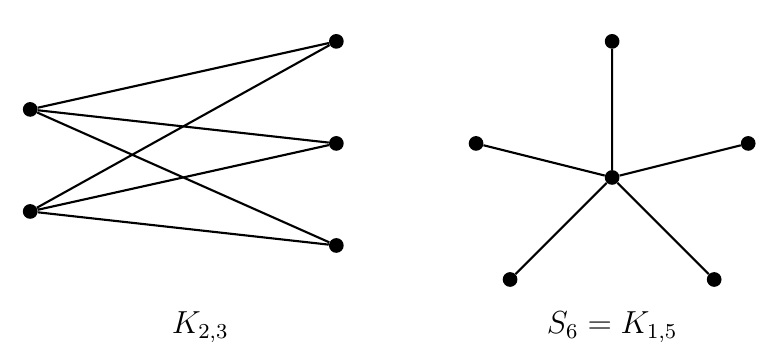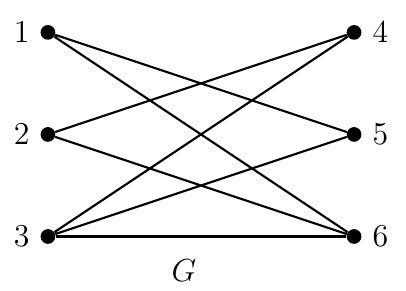Discrete Math Home

## Bipartite Graphs and Matchings

Definition (Bipartite graph). A bipartite graph is a graph whose vertex set can be partitioned into two sets $$V_1$$ and $$V_2$$ (called partite sets) such that each of its edges joins a vertex $$V_1$$ with a vertex of $$V_2$$.Example.

1. $$K_{m,n}$$, the complete bipartite graph on $$m+n$$ vertices, is the simple graph whose vertex set is partitioned into two sets $$V_1$$ and $$V_2$$ such that $$|V_1|=m$$, $$|V_2|=n$$, and each vertex $$V_1$$ is adjacent with each vertex of $$V_2$$. Note that $$|E(K_{m,n})|=mn$$.

2. $$S_n$$, the star on $$n\geq 3$$ vertices, is the complete bipartite graph $$K_{1,n-1}$$.

3. An even cycle, i.e., $$C_n$$ with even $$n$$, is a bipartite graph. But odd cycles are not bipartite graphs.

Definition (Subgraph). A subgraph of a graph $$G=(V,E)$$ is a graph $$H$$ whose vertex set $$V(H)$$ is a subset of $$V$$ and edge set $$E(H)$$ is a subset of $$E$$. A subgraph $$H$$ of $$G$$ is an induced subgraph of $$G$$ induced by the vertex set $$W$$ if its vertex set is $$V(H)=W$$ and edge set $$E(H)$$ is the set of all edges in $$G$$ that have both endpoints in $$V(H)=W$$.

Example. $$K_{1,2}$$ and $$K_{2,2}=C_4$$ are induced subgraphs of $$K_{2,3}$$. $$5K_1$$ and $$2K_2$$ are subgraphs (not induced subgraphs) of $$K_{2,3}$$.

Theorem. A graph is bipartite if and only if it does not contain an odd cycle as a subgraph.

Suppose graph $$G$$ is bipartite with partite sets $$V_1$$ and $$V_2$$. Let cycle $$C_k$$ be a subgraph of $$G$$ with edges $\{x_1,x_2\},\;\{x_2,x_3\},\ldots,\{x_{k-1},x_k\},\;\{x_k,x_1\}.$ Without loss of generality, let $$x_1\in V_1$$. Since $$G$$ is bipartite and $$\{x_1,x_2\}$$ is an edge of $$G$$, $$x_2\in V_2$$. Similarly $$x_i\in V_2$$ for all even $$i\in \{1,2,\ldots,k\}$$. If $$k$$ is odd, then $$x_k\in V_1$$ which (because of the edge $$\{x_k,x_1\}$$) implies $$x_1\in V_2$$, a contradiction. Thus $$k$$ is even and $$C_k$$ is an even cycle. The converse will be proved later with the notion of distance between vertices.

Definition (Matching). A matching of a graph $$G$$ is a set of vertex-disjoint non-loop edges of $$G$$. Each vertex in a matching $$M$$ is called matched by $$M$$ and $$M$$ is said to match each vertex of $$M$$. A matching $$M$$ of $$G$$ is a perfect matching if each vertex of $$G$$ is matched by $$M$$.Example. Each of vertices $$1,3,5,6$$ of the preceding bipartite graph $$G$$ is matched by matchings $$\{\{1,5\},\{3,6\}\}$$ and $$\{\{1,6\},\{3,5\}\}$$. Note that $$\{\{1,5\},\{2,4\},\{3,6\}\}$$ and $$\{\{1,6\},\{2,4\},\{3,5\}\}$$ are perfect matchings of $$G$$

Remark. A graph with a perfect matching has even number of vertices. A necessary and sufficient condition for a graph to have a perfect matching is given by Tutte's theorem in terms of certain connected components of the graph.

Definition (Neighborhood). The neighborhood of a vertex set $$W$$ in a graph $$G$$, denoted by $$\operatorname{N}(W)$$, is the set of all vertices in $$G$$ that are adjacent with some vertex in $$W$$. For a vertex $$v$$ in $$G$$, $$\operatorname{N}(\{v\})$$ is simply denoted by $$\operatorname{N}(v)$$.

Example. In the preceding graph $$G$$, $$\operatorname{N}(1)=\{5,6\}$$ and $$\operatorname{N}(\{1,5\})=\{1,3,5,6\}$$.

Theorem (Hall's marriage theorem, 1935). Let $$G$$ be a bipartite graph with partite sets $$V_1$$ and $$V_2$$. There is a matching $$M$$ of $$G$$ for which each vertex of $$V_1$$ is matched by $$M$$ if and only if for all subsets $$S\subseteq V_1$$, $|S|\leq |N(S)|.$

Suppose there is a matching $$M$$ of $$G$$ for which each vertex of $$V_1$$ is matched by $$M$$. Let $$S\subseteq V_1$$. Each vertex of $$S$$ is adjacent to a vertex in $$V_2$$ via an edge in $$M$$. Since the edges of $$M$$ are vertex-disjoint, $$|S|\leq |N(S)|$$.

Conversely suppose $$|S|\leq |N(S)|$$ for all subsets $$S\subseteq V_1$$. We can find a matching $$M$$ of $$G$$ that matches each vertex of $$V_1$$ by strong induction on $$|V_1|$$ (details skipped).

Remark. To see the preceding theorem in terms of marriage, consider $$m$$ men and $$n$$ women where each of these $$m$$ men has a subset of these $$n$$ women acceptable as a spouse and each of these $$n$$ women would marry a man who wants to marry her. Is it possible that all of the $$m$$ men can be married? The answer is yes if and only if for any subset $$S$$ of men, the number of their possible spouses is bigger than $$|S|$$.

Last edited